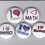# Can bullets and planks madden you? Maybe yes!

A bullet starts with some initial velocity $u$.

It hits a plank which reduces it's velocity by $\dfrac{u}{x}$.

Let $n$ be the number of planks required to stop it.

So on further calculations, I knew that $n = \dfrac{x^2}{2x-1}$

Is it correct? I am unsure as

If $x = 20$

Then $n = 11$ approx.

If $x=50$

Then $n = 26$ approx .

This means that the larger the velocity the plank will decrease, the larger will be the no. of planks required. This is quite weird as it should be the opposite.

Now, this is how I got my formula.

Note: All symbols have their usual meaning like $v , u , a$ and $s$.

Let the no. of planks required be $n$

Let a plank decrease it's velocity $u$ by $\dfrac{u}{x}$

Let thickness of one plank be $z$

After crossing the first plank , $v = u - \dfrac{u}{x}$

Now, by third equation of motion for the first plank,

$v^2 = u^2 + 2as$

$\left(u - \dfrac{u}{x}\right)^2 = u^2 + 2az$

$\left(u - \dfrac{u}{x}\right)^2 - u^2 = 2az$

$\left(\dfrac{ux - u}{x}\right)^2 -u^2 = 2az$

$\dfrac{(ux)^2 + u^2 - 2u^2 x - (ux)^2}{x^2} = 2az$

$\dfrac{u^2 - 2u^2 x}{2x^2 z} = a$

Now after crossing $n$ planks, it's velocity will be 0.

Now by again applying third equation of motion we get,

$v^2 = u^2 + 2as$

Here, $v = 0$

$0 = u^2 + 2 (\dfrac{u^2 - 2u^2 x}{2x^2 z}) (n)(z)$

$0 = u^2 + \dfrac{u^2 - 2u^2 x}{x^2} (n)$

$0 = \dfrac{(ux)^2 + (u^2 - 2u^2 x)n}{x^2}$

$0 = u^2 x^2 + (u^2 - 2u^2 x)n$

$0 = u^2(x^2 + [1 - 2x]n)$

$0 = x^2 + (1 - 2x)n$

$-x^2 = (1 - 2x)n$

$\large\dfrac{-x^2}{1 - 2x} = n$

      OR


$\large n=\dfrac{x^2}{2x - 1}$

I hope that this is correct.Note by Vinayak Bansal
3 years, 5 months ago

This discussion board is a place to discuss our Daily Challenges and the math and science related to those challenges. Explanations are more than just a solution — they should explain the steps and thinking strategies that you used to obtain the solution. Comments should further the discussion of math and science.

When posting on Brilliant:

• Use the emojis to react to an explanation, whether you're congratulating a job well done , or just really confused .
• Ask specific questions about the challenge or the steps in somebody's explanation. Well-posed questions can add a lot to the discussion, but posting "I don't understand!" doesn't help anyone.
• Try to contribute something new to the discussion, whether it is an extension, generalization or other idea related to the challenge.

MarkdownAppears as
*italics* or _italics_ italics
**bold** or __bold__ bold
- bulleted- list
• bulleted
• list
1. numbered2. list
1. numbered
2. list
Note: you must add a full line of space before and after lists for them to show up correctly
paragraph 1paragraph 2

paragraph 1

paragraph 2

[example link](https://brilliant.org)example link
> This is a quote
This is a quote
    # I indented these lines
# 4 spaces, and now they show
# up as a code block.

print "hello world"
# I indented these lines
# 4 spaces, and now they show
# up as a code block.

print "hello world"
MathAppears as
Remember to wrap math in $$ ... $$ or $ ... $ to ensure proper formatting.
2 \times 3 $2 \times 3$
2^{34} $2^{34}$
a_{i-1} $a_{i-1}$
\frac{2}{3} $\frac{2}{3}$
\sqrt{2} $\sqrt{2}$
\sum_{i=1}^3 $\sum_{i=1}^3$
\sin \theta $\sin \theta$
\boxed{123} $\boxed{123}$